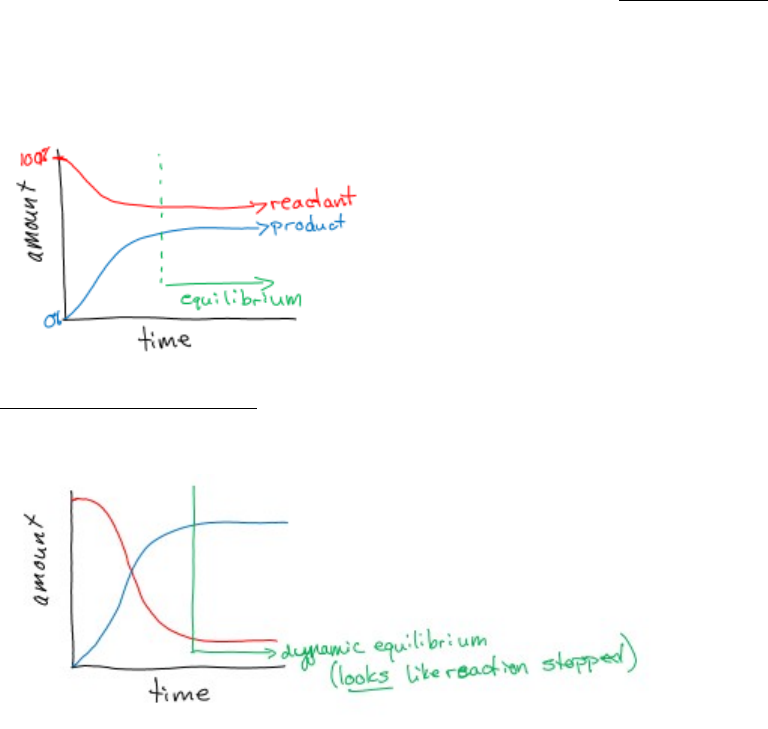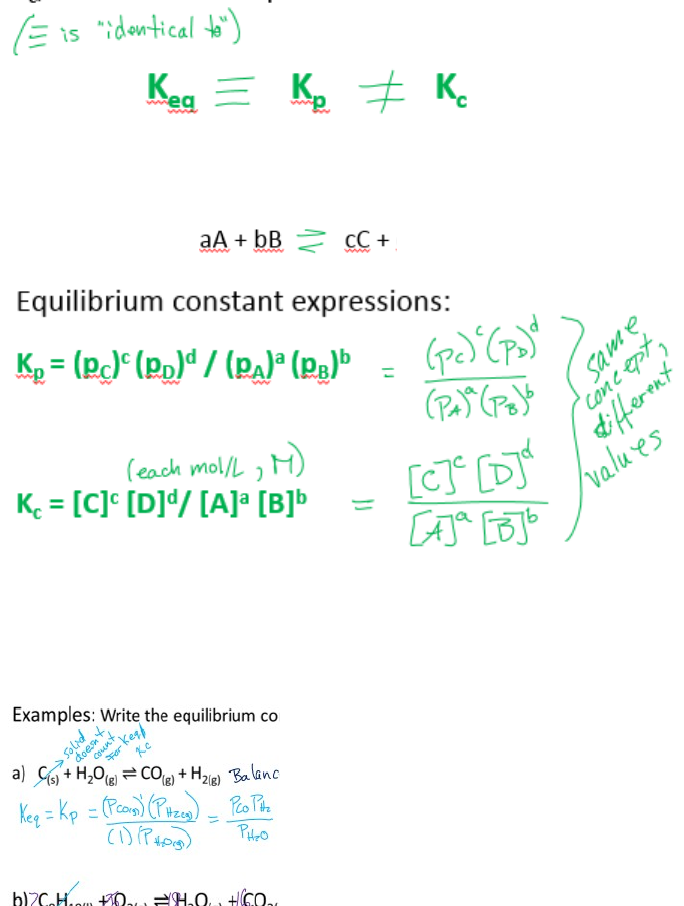Class Notes (1,100,000)
CA (620,000)
Carleton (20,000)
CHEM (200)
Lecture 6

# CHEM 1001 Lecture Notes - Lecture 6: Quadratic Equation, Equilibrium Constant, Stoichiometry

Department
Chemistry
Course Code
CHEM 1001
Professor
Bob Burk
Lecture
6

This preview shows page 1. to view the full 5 pages of the document.Chemistry Topic 6 Equilibrium
In most reactions there is a balance between which direction a reaction goes. A one way
reaction is one that is more likely to go in a certain way. However, for most reactions there is a
balance between the forwards and backwards reactions, called EQUILIBRIUM.
With equilibrium it is likely that some reactions may have the reverse reaction happening at the
exact same time.
As reactants decrease to constant amount, products increase to constant amount
DYNAMIC EQUILIBRIUM: If the rate of forward = rate of backward
This looks like nothing more is happening, the reaction has “stopped”
Equilibrium constant (K(eq)) relates to forward and backward reaction at the same time;
1. Constant for a specific reaction at a given condition
2. Related to the stoichiometry of the balanced equation
oK(eq) = (products)^n/(reactants)^n
oNeed balanced equation for the n values
oProduct and reactant factors in either pressured of concentrations
3. Applies only at equilibrium
4. Independent of initial conditions

Unlock to view full version

Only page 1 are available for preview. Some parts have been intentionally blurred.oThis concept of products to reactants ratio is the same as reaction quotient (Q)
but only applies to the reaction once it has reached equilibrium
oQ applies to any point in the reaction progression
Q = (products)^n/(reactants)^n; when not at equilibrium
The equilibrium constant is determined from either the rate constants or the pressure (or
concentration) conditions of the reaction system.
Note: if K(eq) > 1000, reaction has virtually gone to completion (there is too little left of
reactants)
There are two forms of the equilibrium constant;
K(p), pressure equilibrium constant
K(c), concentration equilibrium constant
The general form;
The phases may be
affected of the species in the reaction matter from this point forward;
Solid or pure liquid does not affect the equilibrium constant (p and or c = 1)
oLeft out of equilibrium equation
Dissolved solids (like aqueous solutions) and gases are
the only phases affecting the equilibrium constant